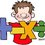# Can someone tell me how to approach and solve this math problem?

If the pair of lines $6x^2-pxy-3y^2-24x+3y+q=0$ intersect on x- axis then p is equal to :- $a)3/2$ $b)-5/2$ $c)-18$ $d)-7$

Please post the solution in DETAIL...Note by Parag Zode
6 years, 5 months ago

This discussion board is a place to discuss our Daily Challenges and the math and science related to those challenges. Explanations are more than just a solution — they should explain the steps and thinking strategies that you used to obtain the solution. Comments should further the discussion of math and science.

When posting on Brilliant:

• Use the emojis to react to an explanation, whether you're congratulating a job well done , or just really confused .
• Ask specific questions about the challenge or the steps in somebody's explanation. Well-posed questions can add a lot to the discussion, but posting "I don't understand!" doesn't help anyone.
• Try to contribute something new to the discussion, whether it is an extension, generalization or other idea related to the challenge.
• Stay on topic — we're all here to learn more about math and science, not to hear about your favorite get-rich-quick scheme or current world events.

MarkdownAppears as
*italics* or _italics_ italics
**bold** or __bold__ bold
- bulleted- list
• bulleted
• list
1. numbered2. list
1. numbered
2. list
Note: you must add a full line of space before and after lists for them to show up correctly
paragraph 1paragraph 2

paragraph 1

paragraph 2

[example link](https://brilliant.org)example link
> This is a quote
This is a quote
    # I indented these lines
# 4 spaces, and now they show
# up as a code block.

print "hello world"
# I indented these lines
# 4 spaces, and now they show
# up as a code block.

print "hello world"
MathAppears as
Remember to wrap math in $$ ... $$ or $ ... $ to ensure proper formatting.
2 \times 3 $2 \times 3$
2^{34} $2^{34}$
a_{i-1} $a_{i-1}$
\frac{2}{3} $\frac{2}{3}$
\sqrt{2} $\sqrt{2}$
\sum_{i=1}^3 $\sum_{i=1}^3$
\sin \theta $\sin \theta$
\boxed{123} $\boxed{123}$

## Comments

Sort by:

Top Newest

For to intersect this pair of straight line on x-axis , Let They intersect at point P ($\alpha \, 0$ )

So putting y=0 in given equation

$6\alpha ^{ 2 }\quad -\quad 24\alpha \quad +\quad q\quad =\quad 0$.

Now since Intersection point is unique so value of $\alpha$ is also unique. So Discriminant of this quadratic equation is zero !!

$q\quad =\quad 24\\ \\ \alpha \quad =\quad 2$.

Therefore Given equation representing pair of straight lines if it's Discriminant is zero !!

$\left| \begin{matrix} 6 & -p/2 & -12 \\ -p/2 & -3 & 3/2 \\ -12 & 3 & 24 \end{matrix} \right| \quad =\quad 0\\ \\ P\quad =\quad 3/2$.

- 6 years, 5 months ago

Log in to reply

Ok! I didn't knew to use determinant after finding q and a(alpha).. Anyways ,Nice solution.

- 6 years, 5 months ago

Log in to reply

The line(s) intersect the x-axis when y=0. The equation then becomes 6x^2 - 24x + q = 0. There are tons of values for x that will solve this equation depending on the value of q.

If y = 0 then values for p are limitless (infinite).

- 6 years, 5 months ago

Log in to reply

Sir but can we assume that for finding value of q the equation$6x^2-24x+q=0$ should be perfect square ? If it is then the value of q is equal to 24. This is a JEE Question .

- 6 years, 5 months ago

Log in to reply

Are there 2 lines? I see only 1 equation.

- 6 years, 5 months ago

Log in to reply

The equation above is multiplication of those 2 lines

- 6 years, 5 months ago

Log in to reply

What are the 2 lines?

- 6 years, 5 months ago

Log in to reply

That is what we have to find in terms of 'p'

- 6 years, 5 months ago

Log in to reply

You also have a q which affects the situation.

If this line intersects the x axis then the value for y = 0.

- 6 years, 5 months ago

Log in to reply

×

Problem Loading...

Note Loading...

Set Loading...